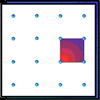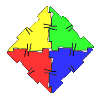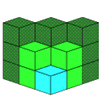You may also likeGeoboards

This practical challenge invites you to investigate the different squares you can make on a square geoboard or pegboard.Polydron

This activity investigates how you might make squares and pentominoes from Polydron.If you had 36 cubes, what different cuboids could you make?

Cover the Tray

Age 7 to 11Challenge Level

Maria and Rosalind from Maryvale USA were the only ones to come up with a solution to the first challenge. This is what they wrote:

First challenge:
To solve this problem, at first we did 18 x 18 which equals 324. The reason we did 18 x 18 is to find the area of the card.
Next, we did 432 - 324.The answer we got was 108.  The 108 really represents 108 cm ².  Also, 108 cm ² represents the difference between the amount of wrapping paper used and the card.

This problem was very hard, and we made many errors before solving it.  At first we started with a drawing (model) of a square.  We labeled the sides 18cm each. The first attempt, we thought, (18 x n) x 4 = the difference 108 cm ².  We divided both sides of the equation by four and found 18n = 27. And that n = 1.5 which can't be true because it can only be whole centimeters.

Second attempt:
Sadly, the second try didn't work either.  We tried solving the problem by realising we DON'T have to wrap the little square of n ².  So the equation was 4 x (18 x n) - 4n ² = 108 cm ².  But that did not give us a whole number either.

Final Try:
We realised that the outside panels aren't 18 cm long anymore because we need to subtract 1n from both ends.  So the equation is: 4 x [(18 - 2n) x n] - 4n ² = 108 cm ². At the end we found 18n - 3n ² = 27. Therefore, our answer for n = 3cm.

The square corners will be 3cm by 3cm, and the dimensions of the base square is 12 cm x 12 cm .

Thank you for the explanation of your attempts and allowing us to see the errors that occurred along the way.